JAJSHO6F March   2005  – July 2019

PRODUCTION DATA.

1. 特長
2. アプリケーション
3. 概要
1.     Device Images
4. 改訂履歴
5. Pin Configuration and Functions
6. Specifications
7. Parameter Measurement Information
8. Detailed Description
9. Application and Implementation
1. 9.1 Application Information
2. 9.2 Typical Applications
1. 9.2.1 Comparator Application
2. 9.2.2 Other Application Circuits
10. 10Power Supply Recommendations
11. 11Layout
12. 12デバイスおよびドキュメントのサポート
13. 13メカニカル、パッケージ、および注文情報

#### パッケージ・オプション

デバイスごとのパッケージ図は、PDF版データシートをご参照ください。

• DBZ|3
• DBV|5

### 6.8 Typical CharacteristicsData is for devices having the indicated value of VREF at IKA = 10 mA, TA = 25°C.
Figure 1. Reference Voltage vs Free-air Temperature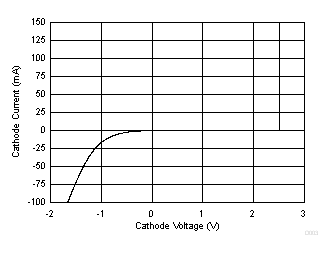Figure 3. Cathode Current vs Cathode VoltageFigure 5. OFF-State Cathode Current vs
Free-air Temperature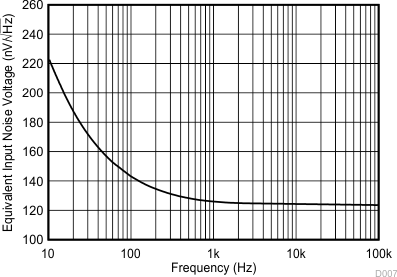Figure 7. Equivalent Input Noise Voltage vs Frequency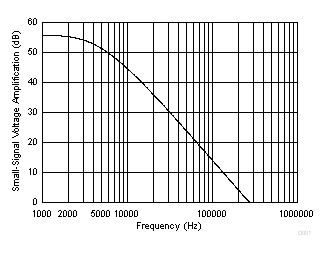Figure 9. Small-Signal Voltage Amplification vs Frequency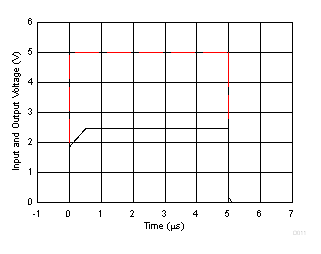Figure 11. Pulse Response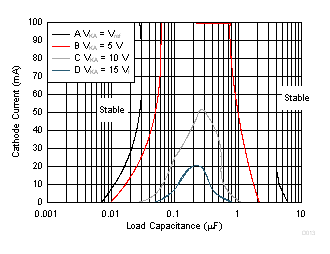The areas under the curves represent conditions that may cause the device to oscillate. For curves B, C, and D, R2 and VREF were adjusted to establish the initial VKA and IKA conditions with CL = 0. VBATT and CL then were adjusted to determine the ranges of stability (see Figure 18 and Figure 19 for test circuits).
Figure 13. Stability Boundary Conditions for All TL43x, SOT-23, SC-70, and Q-TEMP Devices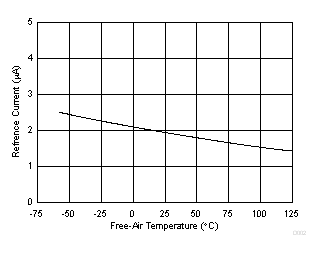Figure 2. Reference Current vs Free-air Temperature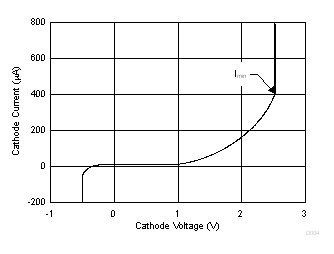Figure 4. Cathode Current vs Cathode Voltage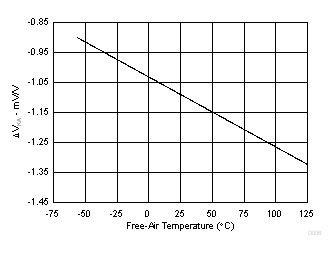Figure 6. Ratio of Delta Reference Voltage to Delta Cathode Voltage vs Free-air Temperature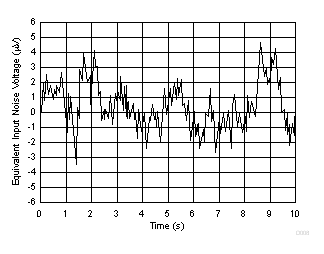Figure 8. Equivalent Input Noise Voltage Over a 10-s Period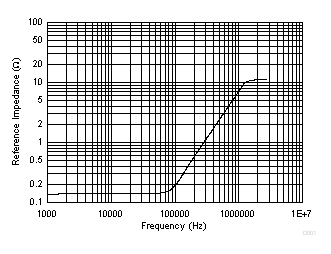Figure 10. Reference Impedance vs Frequency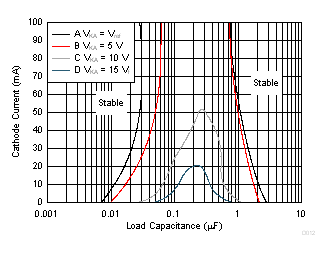The areas under the curves represent conditions that may cause the device to oscillate. For curves B, C, and D, R2 and VREF were adjusted to establish the initial VKA and IKA conditions with CL = 0. VBATT and CL then were adjusted to determine the ranges of stability (see Figure 18 and Figure 19 for test circuits).
Figure 12. Stability Boundary Conditions for All TL43x Devices (Except for SOT23-3, SC-70,
and Q-TEMP Devices)